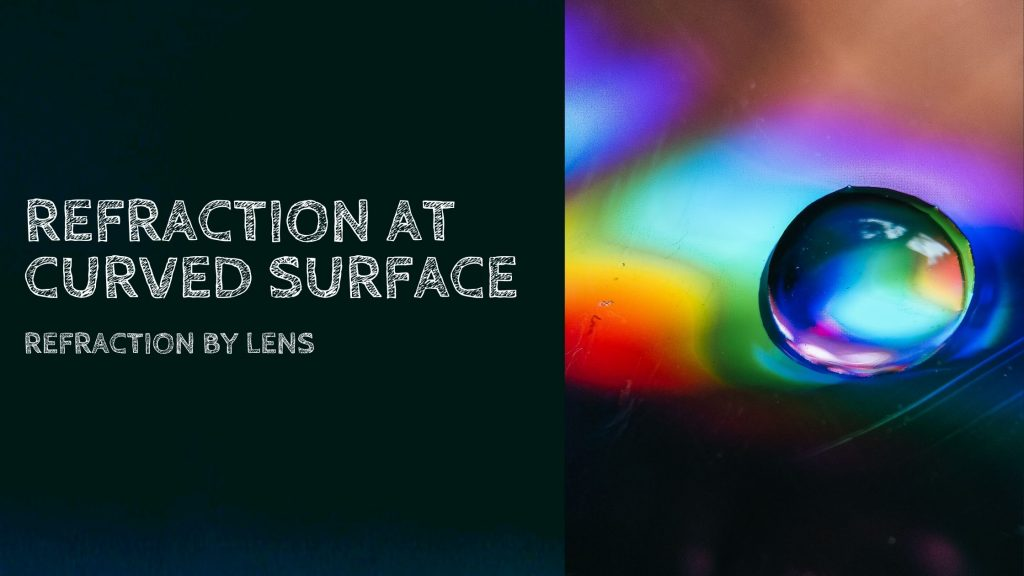Physics
Study Material

# Refraction of Light At Curved Surfaces and By Lens

2 minutes long
Posted by Mahak Jain, 11/8/2021Hesap Oluştur

Got stuck on homework? Get your step-by-step solutions from real tutors in minutes! 24/7. Unlimited.

We have so far considered refraction at a plane interface. We shall now consider refraction of light at curved surfaces between two transparent media. An infinitesimal part of a spherical surface can be regarded as planar and the same laws of refraction can be applied at every point on the surface. Just as for reflection by a spherical mirror, the normal at the point of incidence is perpendicular to the tangent plane to the spherical surface at that point and, therefore, passes through its centre of curvature. We first consider refraction by a single spherical surface and follow it by thin lenses.

A thin lens is a transparent optical medium bounded by two surfaces; at least one of which should be spherical. Applying the formula for image formation by a single spherical surface successively at the two surfaces of a lens, we shall obtain the lens maker’s formula and then the lens formula.

## Refraction of light at a curved surfaces

Figure shows the geometry of formation of image I of an object O on the principal axis of a spherical surface with centre of curvature C, and radius of curvature R. The rays are incident from a medium of refractive index n1 , to another of refractive index n2. As before, we take the aperture (or the lateral size) of the surface to be small compared to other distances involved, so that small angle approximation can be made. In particular, NM will be taken to be nearly equal to the length of the perpendicular from the point N on the principal axis. We have, for small angles.

## Refraction by a lens

Figure shows the geometry of image formation by a double convex lens. The image formation can be seen in terms of two steps:
(i) The first refracting surface forms the image I1 of the object O
[Fig. 9.18(b)]. The image I1 acts as a virtual object for the second surface
that forms the image at I [Fig. 9.18(c)]. Applying Eq. (9.15) to the first
interface ABC, we get.